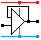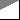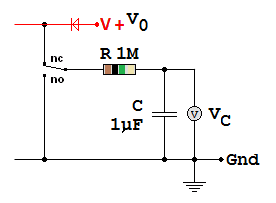Home

rOm
Quest
Glossary

Random
Page
Search
Site
Lush
Sim
Class
Subject
Images

Help
FAQ
Sign
Up
Log
In
 AS Level     Timing     >RC Timing<     Schmitt NOT Astable

# Timing RC Timing

Site for Eduqas/WJEC - Go to the AQA site.

AS Level    RC TimingQuestions 0 to 3   -->  View All

In addition to the basic timing concepts needed for all subject levels, AS and A Level students need a more mathematical approach involving logarithmic and exponential maths. This involves the ln button on your calculator that you may not have used before. The inverse button function is the ex exponential. Some calculators have separate buttons for these functions. You'll also need to learn the difference between the - button and the ± button. In everyday English you may have heard of exponential growth or decay. That's the ex button on your calculator.## Time Constant = R C

Charging:             T63%   =   R C        T50%   =   0.69 R C        T100%   =   5 R C

Discharging:         T37%   =   R C        T50%   =   0.69 R C        T0%   =   5 R C

555 Monostable:   T66.6% =   1.1 R C

## Charging Volts

VC   =   V0 ( 1 - e - t / RC)    If you know the time, you can calculate the volts.

## Discharging Volts

VC   =   V0 e - t / RC    If you know the time, you can calculate the volts.

## Charging Time

t = -RC ln( 1 - VC / V0 )    If you know the volts, you can calculate the time.

## Discharging Time

t = -RC ln( VC / V0 )    If you know the volts, you can calculate the time.

## RC Timing Circuit

• The power supply voltage is V0
• The capacitor voltage is VC
• The elapsed time is t.For this circuit, the time constant   T   =   R C   =   1 x 106 x 1 x 10-6   =   1 Second

For an uncharged capacitor, after a time t, the capacitor voltage   VC   =   V0 ( 1 - e - t / RC)

This is tricky to work out with a calculator. You could attempt it using loads of brackets.

Or you could break it down into smaller safer calculations.

1. Work out   t / (R C)
2. Work out   e-( answer from step 1 )
3. Work out   1   -   (answer from step 2)
4. Work out   V0   x   (answer from step 3)

Here is a test case. After 0.69 seconds, the circuit above should have charged to 50% of V0. If V0 is 100 Volts, the answer should be very close to 50V.

1. Work out   t / (R C)   =   0.69 / ( 1 x 1 ) because the Megohm 106 cancels out with the microfarad 10-6   =   0.69.
2. Work out   e-( answer from step 1 )   =   e -0.69   =   0.502
3. Work out   1   -   (answer from step 2)   =   1 - 0.502   =   0.498
4. Work out   V0   x   (answer from step 3)   =   49.8 Volts   Very close to the expected answer - less than 1% out.

## New Calculator Skills Video

Subject Name     Level     Topic Name     Question Heading     First Name Last Name Class ID     User ID

 CloseEscapeX Q: qNum of last_q     Q ID: Question ID         Score: num correct/num attempts         Date Done

Question Text

image url

Help Text
Debug

• You can attempt a question as many times as you like.
• If you are logged in, your first attempt, each day, is logged.
• To improve your scores, come back on future days, log in and re-do the questions that caused you problems.
• In the grade book, you can delete your answers for a topic before re-doing the questions. Avoid deleting unless you intend re-doing the questions very soon.